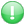Get premium membership and access revision papers, questions with answers as well as video lessons.

# Epsc 224 :Statistics In Behavioural Science  Question Paper

Epsc 224 :Statistics In Behavioural Science

Course:Economics And Statistics

Institution: Laikipia University question papers

Exam Year:2015

LAIKIPIA UNIVERSITY
UNIVERSITY EXAMINATIONS
EPSC 224 :STATISTICS IN BEHAVIOURAL
SCIENCE.

1.a)Define the following terms
I. Descriptive statistics
II. Inferential statistics
III. Variable
IV. Population
V. Sample

1b)Explain using an example the four scales of measurement.

Use the following data given below to answer the questions that follows
68 53 62 61 58 58 57 56 54 54 46 52 52 51 51 50 49 48 47 43 46 45 45 45 45 44 43 43 63 42 42 41 41 41 40 39 39 36 38 37 37 36 35 33 32 30 26 26 21

I. Prepare a frequency distribution showing the class stated and real limits, cumulative frequency and midpoints. Use i=7
II. Find the range, median and mean
III. Prepare a polygon for the above data
IV. Give two merits of Using mode as a measure of central tendency
V. Calculate the variance and standard deviation for the grouped data {show your working}

3.Determine the nature of the linear relationship in the performance of 10 students in two tests as follows
Test 1:78 90 25 30 56 70 69 45 75 60
Test2:79 88 50 45 60 65 75 50 70 59

4.What is the Z score of a score of 70 in a distribution whose mean is 75 and standard deviation is 10
5.State the appropriate situation where the following statistics are used
I. ANOVA
II. T-distribution
III. Chi-square